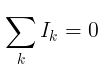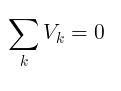# Kirchhoff’s Laws

Kirchhoff's circuit laws are two equalities that deal with the current and potential difference in the lumped element model of electrical circuits. This generalized the work of Georg Ohm and preceded the work of Maxwell. Widely used in electrical engineering, they are also called Kirchhoff's rules or simply Kirchhoff's laws. two of the most important DC network principles involve currents that flow into and out of specific circuit points, and the sums of the voltages around closed loops.

Kirchhoff's Current Law (KCL)

Kirchhoff's Voltage Law (KVL)

#### Kirchhoff's Current Law (KCL)

The sum of all currents that enter an electrical circuit junction is 0. When the currents enter the junction have positive sign and the current that leave the junction have negative sign .#### Kirchhoff's Voltage Law (KVL)

The sum of all voltages or potential differences in an electrical circuit loop is 0.